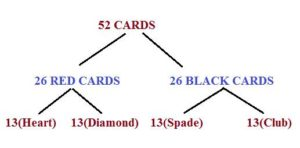# PROBLEMS ON PROBABILITY-HOW TO SERIES

Hi Bankersdaily Aspirants,

How is your Preparation going on?Do well in the exam be calm and Patient during the exam.As always I say Try ,Try Correct Your Mistake & Try until you succeed.But I am Expecting my Aspirants to Try & Try not only the exams but also Try & Practice before the exam so that you will succeed in your Exam.Aspirants Boost up as you all know Motivation will not come from Outside by Just seeing all the Motivational Video’s but it should be stemmed within yourself So that you will definitely Rock in the exam.

### INTRODUCTION:

Today I came with one of the easiest topic in Aptitude Section that is PROBABILITY.This topic is asked for 1 Mark or 2 Mark in Prelims Examination & the Problem from this section will be quite easy & we can solve in a minute.

To Crack the Exam the first thing we should know before knowing how to solve the question is selection of the question.It is important to choose the question which can be solved easily.So,Probability is that kind of Section in which we can solve the question in few seconds.It is wise to attempt the questions from Probability because they can’t trick you after a certain extent.

There is only 1 Rule in Probability that Number of Possible ways/Total Number of Sample Space

1.Based on Coins

2.Based on Dice

3.Based on Cards

## #.1 TYPE 1:

### PROBLEMS BASED ON COINS:

The Possible Number of Outcomes is always Calculated by 2∧n

When a Coin is Tossed then the Possible ways=2¹=2(Head or Tail)

When Three Coins are Tossed then the Possible ways=2³=8(HHH,HHT,HTH,HTT,THH,THT,TTH,TTT)

The Question from this Coin Pattern May have 3 words to trick you that is

ATMOST

ATLEAST

EXACTLY

If in the Question they specify Atmost 2 heads then they are saying that Maximum of two heads

If they Specify Atleast 2 heads then they are saying there should Minimum of Two heads There May be more than 2 heads

If they Specify Exactly 2 heads then there should be only 2 heads not more than that not less than that.

## #.2.TYPE 2:

### PROBLEMS BASED ON DICE:

The same Logic Applies here that is the Possible number of Outcomes is 2 Power n

When a Single Dice is thrown the number of Possibility=6¹=6(1,2,3,4,5,6)

When two Dice are thrown then the number of Possible Outcomes=6²=36

[(1,1)(1,2)(1,3)(1,4)(1,5)(1,6)

(2,1)(2,2)(2,3)(2,4)(2,5)(2,6)

(3,1)(3,2)(3,3)(3,4)(3,5)(3,6)

(4,1)(4,2)(4,3)(4,4)(4,5)(4,6)

(5,1)(5,2)(5,3)(5,4)(5,5)(5,6)

(6,1)(6,2)(6,3)(6,4)(6,5)(6,6)]

## #.3.TYPE 3

### PROBLEMS BASED ON CARDS:

In Card Based Problem Remember this Hierarchy13 Cards in Each Category is:

Numbers (1 to 9) 9 cards

Ace-1,Jack-1 Queen-1 King-1 (4 cards)

### Let us look into Problems from Each Type:

1)What is the Probability of getting Exactly 1 Tail when Three Dice are thrown?

EXPLANATION

The Total number of Possibility=2³=8(HHH,HHT,HTH,HTT,THH,THT,TTH,TTT)

So getting Exactly One Tail={(HHT),(HTH),(THH)}

Probability=Possible Outcomes/Total number of Possibility

Probability=3/8

2)When two dice are thrown at a random Find what is the Probability of Same number in each dice?

EXPLANATION

Same Dice Number of events={(1,1)(2,2)(3,3)(4,4)(5,5)(6,6)}=6

Total Events=36

Probability=6/36=1/6 ways

3)What is the Probability of getting sum greater than 6 when two dice are thrown?

EXPLANATION

Sum greater than 6 when two dice are={(1,6)(2,5)(2,6)(3,4)(3,5)(3,6)(4,3)(4,4)(4,5)(4,6)(5,2)(5,3)(5,4)(5,5)(5,6)(6,1)(6,2)(6,3)(6,4)(6,5)(6,6)}

=21/36=7/12 ways

4)When 2 cards are drawn at Random,what is the Probability that Both the cards are Ace?

EXPLANATION

Both the Card are Ace:

Total number of ways=52C2=52*51/1*2=26*51

Number of Possible Outcomes:

Total number of Ace =1*4=4 cards

From this 2 cards has to be drawn out=4C2=4*3/1*2=6

Therefore,

Possible Outcomes=6/26*51=1/221

Friends we will see more number of questions & how to solve them on Probability in the next Article of Probability.

If you have any query let us know us by Posting your query in the Comment Box